# Many-electron-atoms.ppt

26. Oct 2022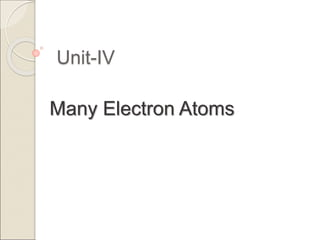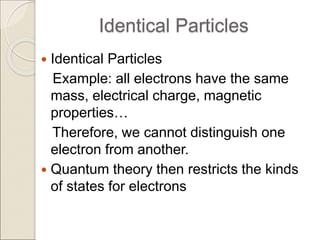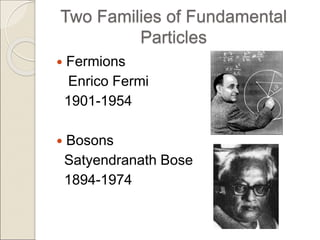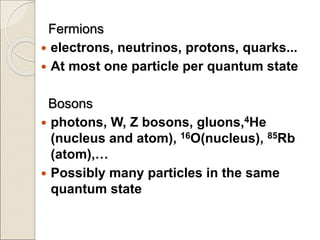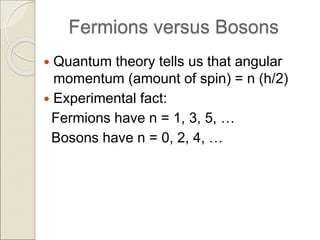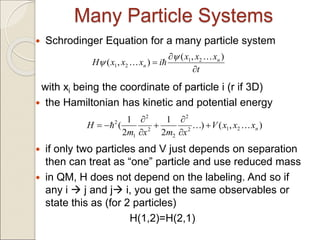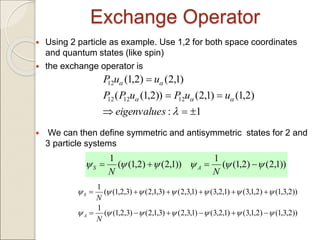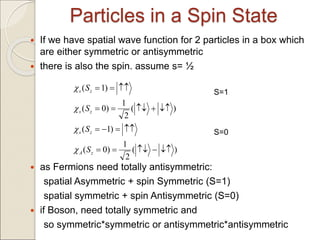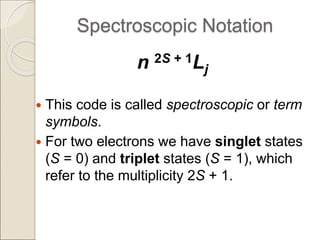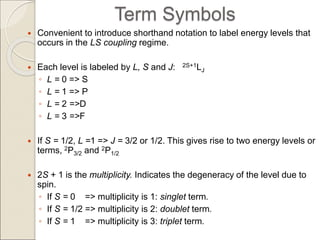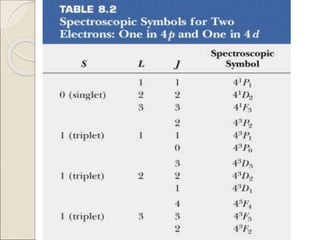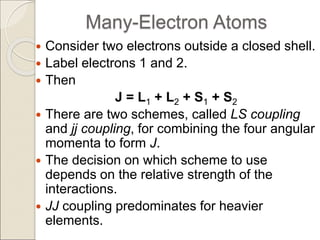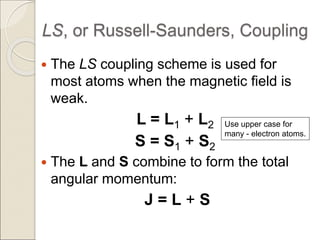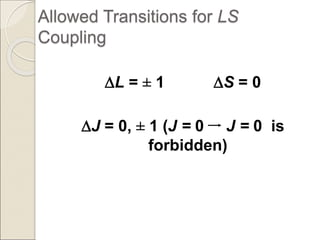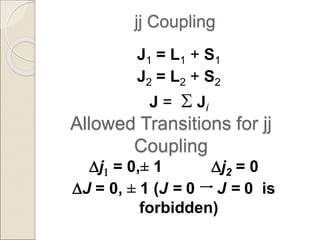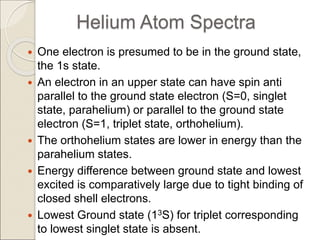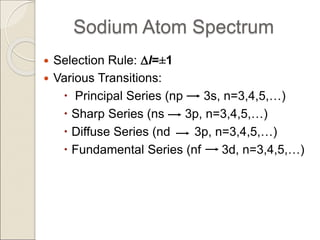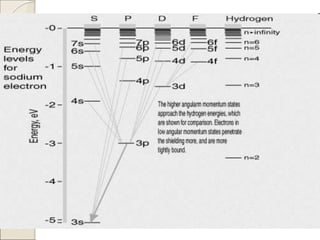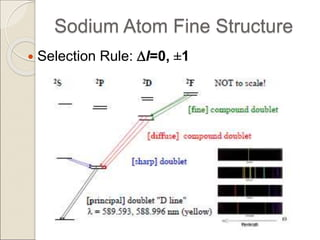1 von 20

### Many-electron-atoms.ppt

• 1. Unit-IV Many Electron Atoms
• 2. Identical Particles  Identical Particles Example: all electrons have the same mass, electrical charge, magnetic properties… Therefore, we cannot distinguish one electron from another.  Quantum theory then restricts the kinds of states for electrons
• 3. Two Families of Fundamental Particles  Fermions Enrico Fermi 1901-1954  Bosons Satyendranath Bose 1894-1974
• 4. Fermions  electrons, neutrinos, protons, quarks...  At most one particle per quantum state Bosons  photons, W, Z bosons, gluons,4He (nucleus and atom), 16O(nucleus), 85Rb (atom),…  Possibly many particles in the same quantum state
• 5. Fermions versus Bosons  Quantum theory tells us that angular momentum (amount of spin) = n (h/2)  Experimental fact: Fermions have n = 1, 3, 5, … Bosons have n = 0, 2, 4, …
• 6. Many Particle Systems  Schrodinger Equation for a many particle system with xi being the coordinate of particle i (r if 3D)  the Hamiltonian has kinetic and potential energy  if only two particles and V just depends on separation then can treat as “one” particle and use reduced mass  in QM, H does not depend on the labeling. And so if any i  j and j i, you get the same observables or state this as (for 2 particles) H(1,2)=H(2,1) t x x x i x x x H n n    ) , ( ) , ( 2 1 2 1      ) , ( ) 2 1 2 1 ( 2 1 2 2 2 2 2 1 2 n x x x V x m x m H           
• 7. Exchange Operator  Using 2 particle as example. Use 1,2 for both space coordinates and quantum states (like spin)  the exchange operator is  We can then define symmetric and antisymmetric states for 2 and 3 particle systems 1 : ) 2 , 1 ( ) 1 , 2 ( )) 2 , 1 ( ( ) 1 , 2 ( ) 2 , 1 ( 12 12 12 12             s eigenvalue u u P u P P u u P )) 1 , 2 ( ) 2 , 1 ( ( 1 )) 1 , 2 ( ) 2 , 1 ( ( 1           N N A S )) 2 , 3 , 1 ( ) 2 , 1 , 3 ( ) 1 , 2 , 3 ( ) 1 , 3 , 2 ( ) 3 , 1 , 2 ( ) 3 , 2 , 1 ( ( 1 )) 2 , 3 , 1 ( ) 2 , 1 , 3 ( ) 1 , 2 , 3 ( ) 1 , 3 , 2 ( ) 3 , 1 , 2 ( ) 3 , 2 , 1 ( ( 1                           N N A S
• 8. Particles in a Spin State  If we have spatial wave function for 2 particles in a box which are either symmetric or antisymmetric  there is also the spin. assume s= ½  as Fermions need totally antisymmetric: spatial Asymmetric + spin Symmetric (S=1) spatial symmetric + spin Antisymmetric (S=0)  if Boson, need totally symmetric and so symmetric*symmetric or antisymmetric*antisymmetric ) ( 2 1 ) 0 ( ) 1 ( ) ( 2 1 ) 0 ( ) 1 (                  z A z s z s z s S S S S     S=1 S=0
• 9. Spectroscopic Notation n 2S + 1Lj  This code is called spectroscopic or term symbols.  For two electrons we have singlet states (S = 0) and triplet states (S = 1), which refer to the multiplicity 2S + 1.
• 10. Term Symbols  Convenient to introduce shorthand notation to label energy levels that occurs in the LS coupling regime.  Each level is labeled by L, S and J: 2S+1LJ ◦ L = 0 => S ◦ L = 1 => P ◦ L = 2 =>D ◦ L = 3 =>F  If S = 1/2, L =1 => J = 3/2 or 1/2. This gives rise to two energy levels or terms, 2P3/2 and 2P1/2  2S + 1 is the multiplicity. Indicates the degeneracy of the level due to spin. ◦ If S = 0 => multiplicity is 1: singlet term. ◦ If S = 1/2 => multiplicity is 2: doublet term. ◦ If S = 1 => multiplicity is 3: triplet term.
• 12. Many-Electron Atoms  Consider two electrons outside a closed shell.  Label electrons 1 and 2.  Then J = L1 + L2 + S1 + S2  There are two schemes, called LS coupling and jj coupling, for combining the four angular momenta to form J.  The decision on which scheme to use depends on the relative strength of the interactions.  JJ coupling predominates for heavier elements.
• 13. LS, or Russell-Saunders, Coupling  The LS coupling scheme is used for most atoms when the magnetic field is weak. L = L1 + L2 S = S1 + S2  The L and S combine to form the total angular momentum: J = L + S Use upper case for many - electron atoms.
• 14. Allowed Transitions for LS Coupling DL = ± 1 DS = 0 DJ = 0, ± 1 (J = 0 J = 0 is forbidden)
• 15. jj Coupling J1 = L1 + S1 J2 = L2 + S2 J = S Ji Allowed Transitions for jj Coupling Dj1 = 0,± 1 Dj2 = 0 DJ = 0, ± 1 (J = 0 J = 0 is forbidden)
• 16. Helium Atom Spectra  One electron is presumed to be in the ground state, the 1s state.  An electron in an upper state can have spin anti parallel to the ground state electron (S=0, singlet state, parahelium) or parallel to the ground state electron (S=1, triplet state, orthohelium).  The orthohelium states are lower in energy than the parahelium states.  Energy difference between ground state and lowest excited is comparatively large due to tight binding of closed shell electrons.  Lowest Ground state (13S) for triplet corresponding to lowest singlet state is absent.
• 17. DL=±1, DS = 0, DJ =0, ± 1
• 18. Sodium Atom Spectrum  Selection Rule: Dl=±1  Various Transitions:  Principal Series (np 3s, n=3,4,5,…)  Sharp Series (ns 3p, n=3,4,5,…)  Diffuse Series (nd 3p, n=3,4,5,…)  Fundamental Series (nf 3d, n=3,4,5,…)
• 20. Sodium Atom Fine Structure  Selection Rule: Dl=0, ±1